#Algebra 2 Worksheets

## Systems of Equations and Inequalities Worksheets

Here is a graphic preview for all of the Systems of Equations and Inequalities Worksheets. You can select different variables to customize these Systems of Equations and Inequalities Worksheets for your needs. The Systems of Equations and Inequalities Worksheets are randomly created and will never repeat so you have an endless supply of quality Systems of Equations and Inequalities Worksheets to use in the classroom or at home. We have handout for systems of equations, solving algebraically two variable systems of equations, solving graphically two variable systems of equations, systems of two linear inequalities, points in three dimensions, planes, systems of three equations, Cramer's rule and systems of two equations word problems for your use.

Our Sequences and Series Worksheets are free to download, easy to use, and very flexible.

These Systems of Equations and Inequalities Worksheets are a good resource for students in the 8th Grade through the 12th Grade.

Click here for a Detailed Description of all the Systems of Equations and Inequalities Worksheets.

## Quick Link for All Systems of Equations and Inequalities Worksheets

Click the image to be taken to that Systems of Equations and Inequalities Worksheets.

##### Handout forSystems of Equations##### Solving AlgebraicallyTwo VariableSystems of Equations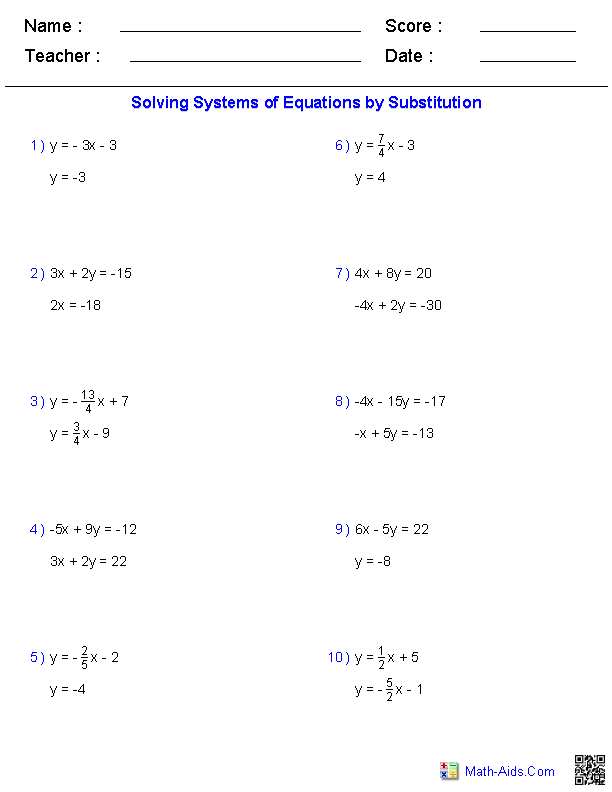##### Solving GraphicallyTwo VariableSystems of Equations##### Solving GraphicallyTwo VariableSystems of Inequalities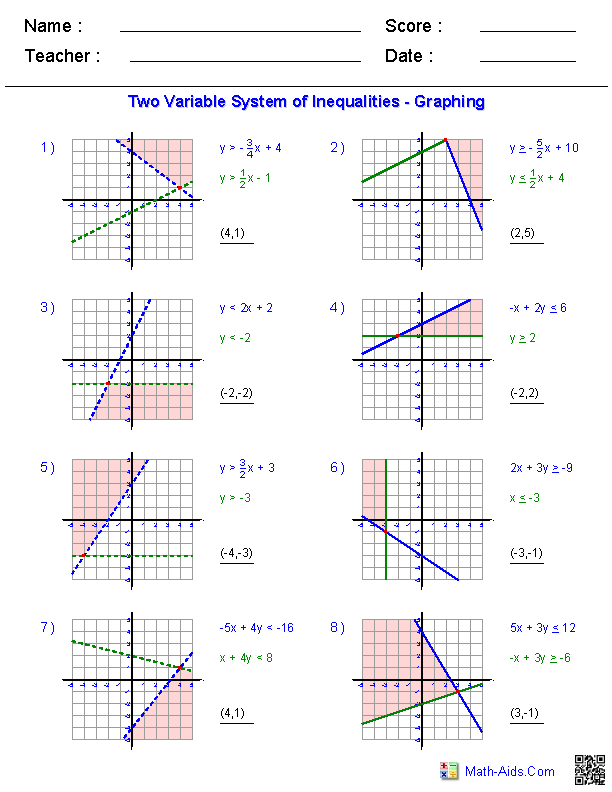##### Systems ofThree Equations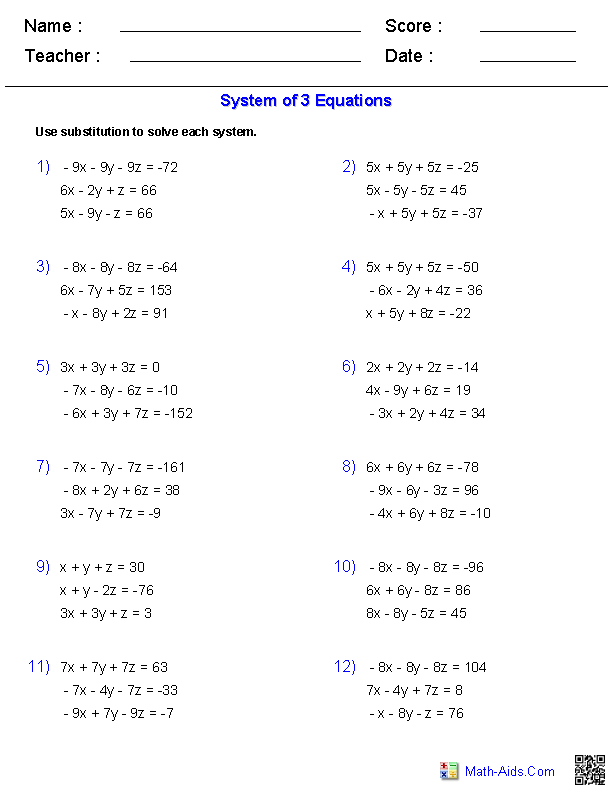##### Systems of Two EquationsWord Problems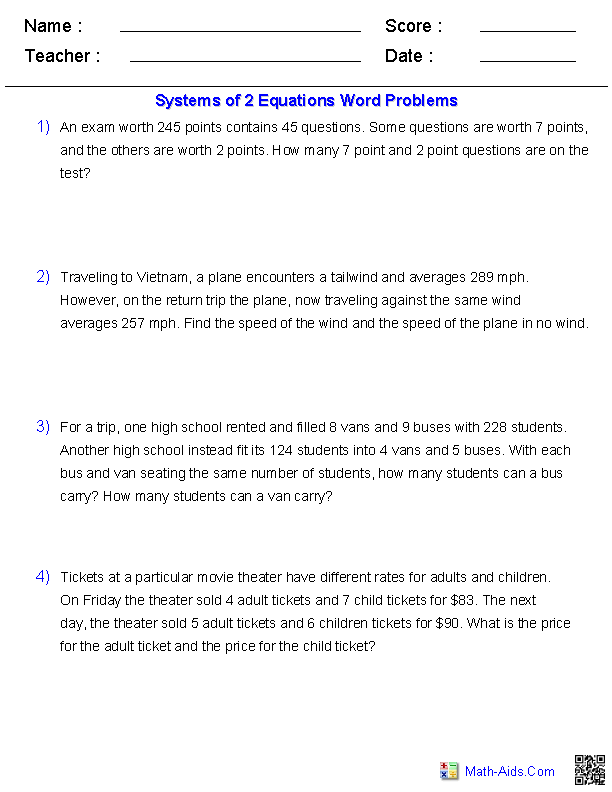##### Systems of Inequalities Multiple Choice Problems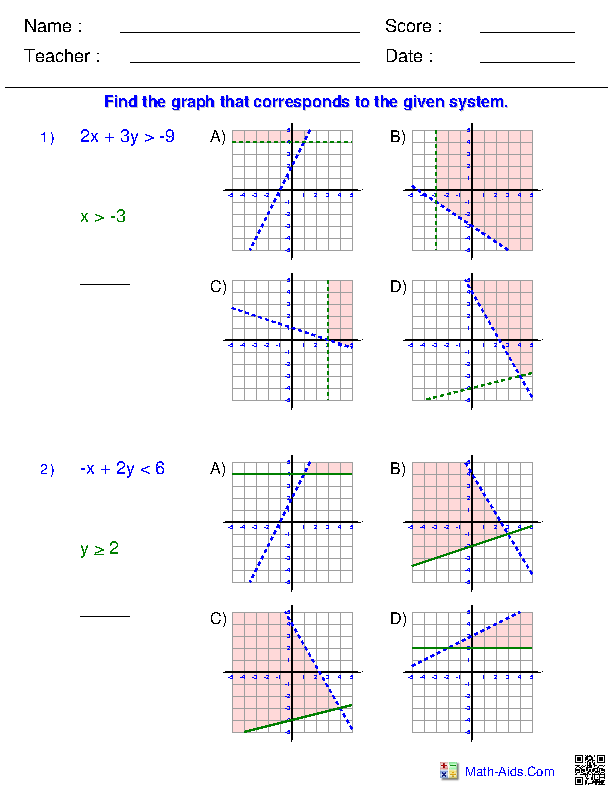##### Cramer's Rule 2x2Matrices Worksheets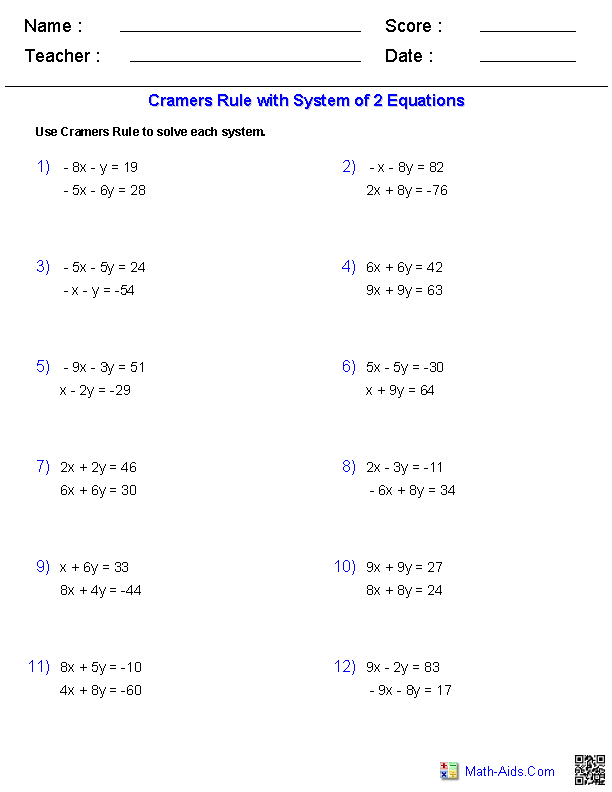##### Cramer's Rule 3x3Matrices Worksheets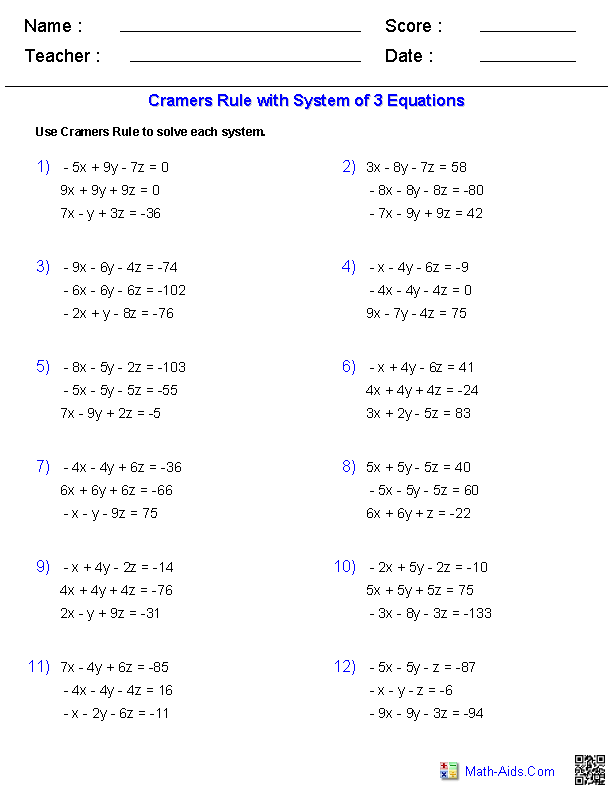Recommended Videos

## Detailed Description for All Systems of Equations and Inequalities Worksheets

Handout for Systems of Equations Worksheets
This Systems of Equations worksheet will produce a handout for the students concerning Systems of Equations. You may select which methods will be produced for the handout. This Systems of Equations worksheet is a good resource for students in the 9th Grade, 10th Grade, 11th Grade, and 12th Grade.

Solving Algebraically Two Variable Systems of Equations Worksheets
This Systems of Equations worksheet will produce problems for solving two variable systems of equations algebraically. You may select which type of method the student should use to solve the problems, as well as the types of solutions. This Systems of Equations worksheet will produce twelve problems per page. This Systems of Equations worksheet is a good resource for students in the 9th Grade, 10th Grade, 11th Grade, and 12th Grade.

Solving Graphically Two Variable Systems of Equations Worksheets
This Systems of Equations worksheet will produce problems for solving two variable systems of equations algebraically. You may select which type of method the student should use to solve the problems, as well as the types of solutions. This Systems of Equations worksheet will produce eight problems per page. This Systems of Equations worksheet is a good resource for students in the 9th Grade, 10th Grade, 11th Grade, and 12th Grade.

Solving Graphically Two Variable Systems of Inequalities Worksheets
This Systems of Equations worksheet will produce problems for solving two variable systems of inequalities graphically. You may select which type of method the student should use to solve the problems. This Systems of Equations worksheet will produce eight problems per page. This Systems of Equations worksheet is a good resource for students in the 9th Grade, 10th Grade, 11th Grade, and 12th Grade.

Systems of Three Equations Worksheets
This Systems of Equations worksheet will produce problems for working with systems of three equations. You can select the method with which to solve the systems as well as the types of solutions. This Systems of Equations worksheet will produce twelve problems per page. This Systems of Equations worksheet is a good resource for students in the 9th Grade, 10th Grade, 11th Grade, and 12th Grade.

Systems of 2 Equations Word Problems Worksheets
This Systems of Equations worksheet will produce problems for working with systems of 2 equations word problems. This Systems of Equations worksheet will produce four problems per page. This Systems of Equations worksheet is a good resource for students in the 9th Grade, 10th Grade, 11th Grade, and 12th Grade.

Systems of Inequalities Multiple Choice Problems
This Systems of Equations worksheet will produce multiple choice problems for solving two variable systems of inequalities graphically. You can select the types of problems and number of problems. This Systems of Equations worksheet will produce two problems per page. This Systems of Equations worksheet is a good resource for students in the 9th Grade, 10th Grade, 11th Grade, and 12th Grade.

Cramer's Rule 2x2 Matrices Worksheets
This Systems of Equations worksheet will produce problems for using Cramer's rule with 2x2 matrices. You can select the types of solutions you want the problems to have. This Systems of Equations worksheet will produce twelve problems per page. This Systems of Equations worksheet is a good resource for students in the 9th Grade, 10th Grade, 11th Grade, and 12th Grade.

Cramer's Rule 3x3 Matrices Worksheets
This Systems of Equations worksheet will produce problems for using Cramer's rule with 3x3 matrices. You can select the types of solutions you want the problems to have. This Systems of Equations worksheet will produce twelve problems per page. This Systems of Equations worksheet is a good resource for students in the 9th Grade, 10th Grade, 11th Grade, and 12th Grade.